共有10317人关注过本帖树形打印复制链接

# 主题：程序代码――输出素数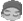1楼 博客 | 信息 | 搜索 | 邮箱 | 主页 | UC加好友发短信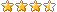管理员

 public class Exec {        public static void main(String args[]) {                for (int i = 1; i <= 1000000; i += 2) {                        boolean flag = true;                         for (int j = 2; j < Math.sqrt(i); j++) {                                if (i % j == 0) {                                        flag = false;                                        break;                                }                        }                         if (flag)                                System.out.println(i);                }         }}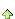20-aini
2楼 博客 | 信息 | 搜索 | 邮箱 | 主页 | UC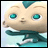加好友发短信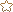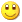Post By：2011/3/14 17:09:04 [只看该作者]

 public class Esce { public static void main(String[] args) {  int i = 1, j = 2;  for (i = 1; i < 101; i++) {   for (j = 2; j < i; j++)    if (i % j == 0) {     break;    } else     System.out.print(i + " ");   } } }为什么把  else  换成   if（i==j） 才对？     if（i==j）在这起什么作用？？加好友发短信管理员